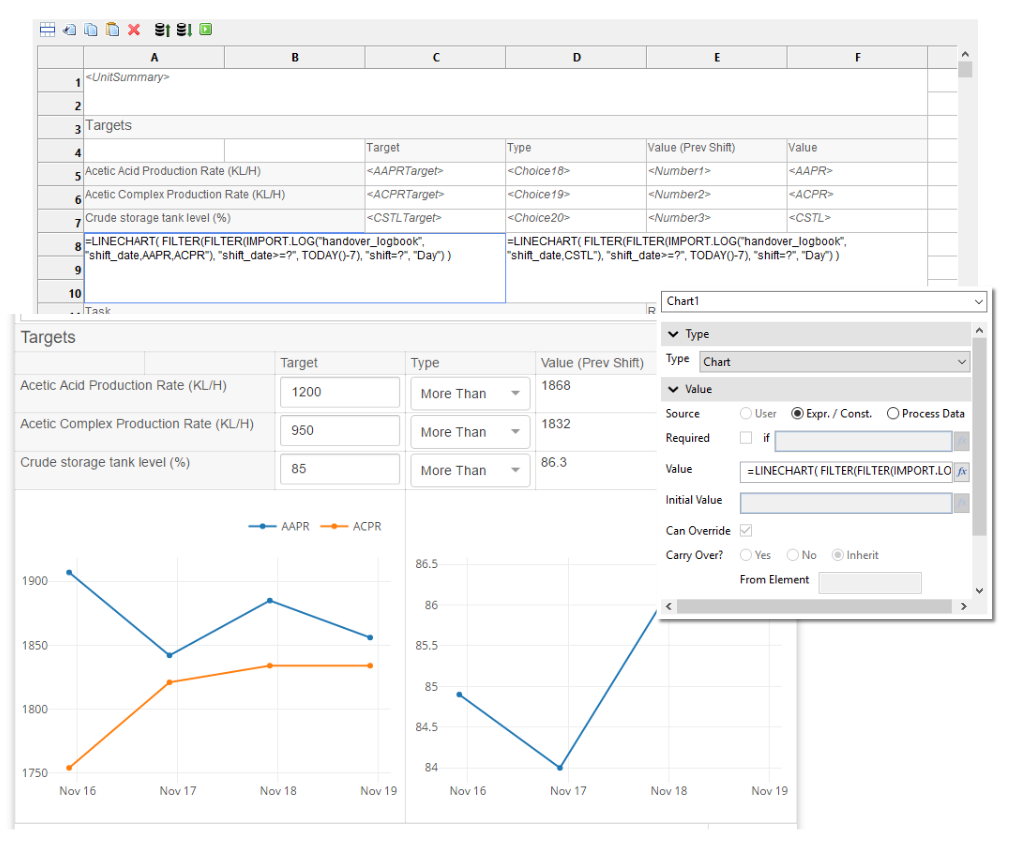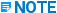# Charts - j5 - 28.0 - Customization & Programming - Hexagon

## j5 IndustraForm Designer Help

Language
English
Product
j5
Search by Category
Customization & Programming
j5 Version
2019

In the last chapter, we added a table with a trend of the KPIs for Unit 1. Now, let’s turn that in to some line charts, instead of a table.

The formula for the Data Binding was as follows:

=FILTER(IMPORT.LOG("handover_logbook",

"shift_date,shift,AAPRTarget,AAPR,ACPRTarget,ACPR,CSTLTarget,CSTL"), "shift_date>=?", TODAY()7)

For our charts, we’re just going to show the values, not the targets, and we’re going to show the CSTL value in a different chart (since its scale is quite different). Finally, we’ll add an extra FILTER to only take the Day shift value so that we can use the shift_date on the x-axis with just one value per day.

This is the formula for the AAPR / ACPR chart:

=LINECHART(FILTER(FILTER(IMPORT.LOG("handover_logbook", "shift_date,AAPR,ACPR"),

"shift_date>=?", TODAY()-7), "shift=?", "Day"))

As you can see, we simply pass the result of a data import formula in to the LINECHART function. This function can be used as the value of a Chart element type, with a value source of Expr. / Const., as shown below. The first column will be used on the x-axis, and the rest of the columns are the y-axis series.The chart axis scale is determined automatically based on the range of values in the chart. The series labels come from the column names in the imported dataset.

See the IndustraForm YML file here: Step 7.It is also possible to draw a BARCHART. This is demonstrated here.